## Solving Proportions With The Toronto Maple Leafs Win:Loss Ratio

As we began the Proportional Reasoning unit in my MFM1P Grade 9 Math Course, I was beginning to struggle with an idea to extend the idea of visualizing mathematics concepts into ratios, rates, and proportions. What I came up with was using a visual representation of Toronto Maple Leaf wins and losses in order to help scaffold students slowly from concrete examples to the algebraic representation utilizing a proportion of equivalent fractions. This could be a good way for you to understand the rankings and wins and losses of your fantasy hockey teams.

If you’re not into watching the video, you can see some screenshots below.

## Summary of the Working With Ratios and Proportions Video

The video begins with a formal definition of a ratio and heads straight into the scenario where the Toronto Maple Leafs win:loss ratio is 3:1.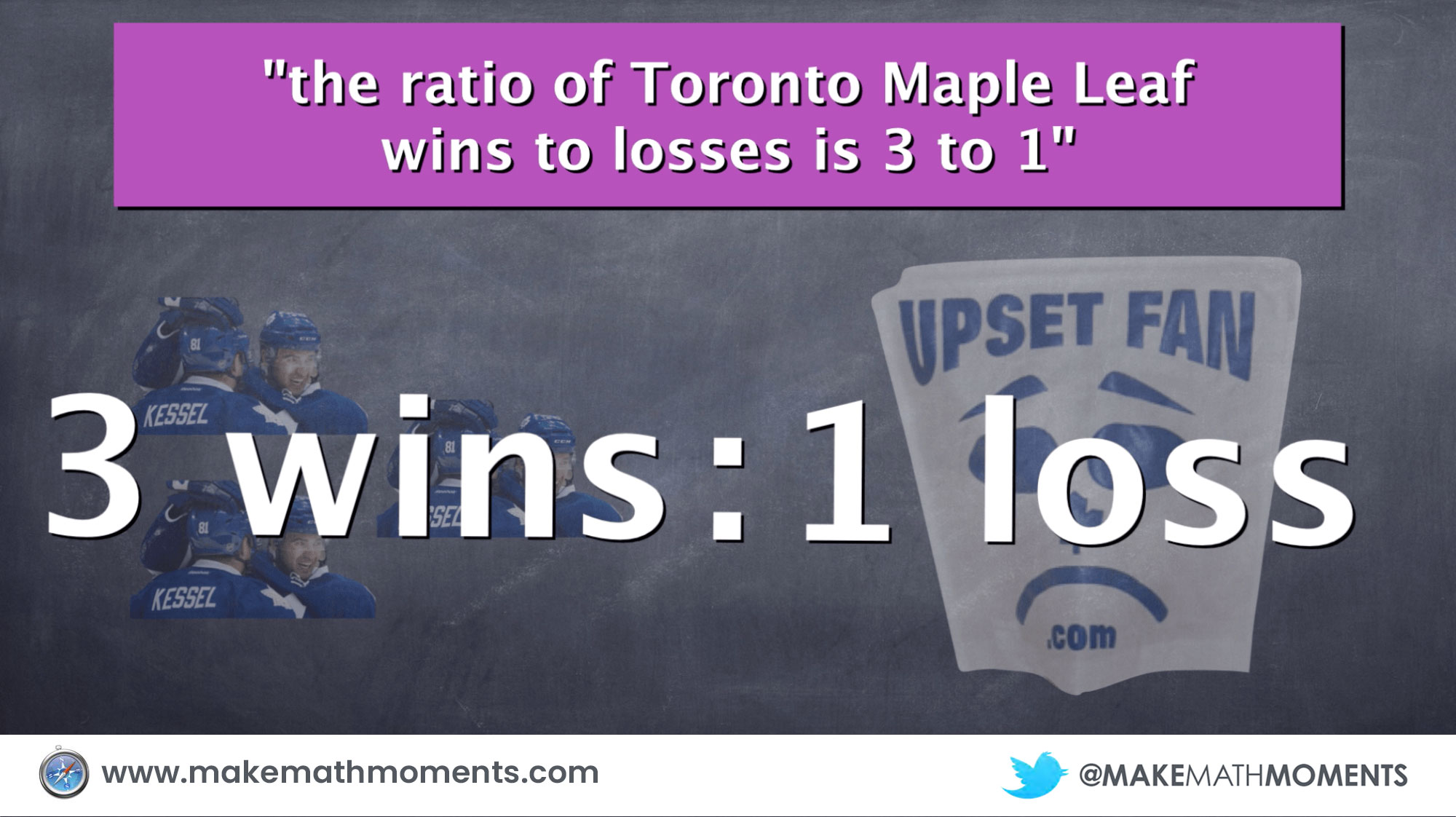Because this is a relatively basic example, many can find the unknown in the proportion using trial and error or even just logic without really understanding what they did to get there. I find it useful to demonstrate that we can see the same 3:1 ratio, 3 times on the right side of the proportion. Using terminology like “3 groups of 3 wins” and “3 groups of 1 loss” can help scaffold towards more difficult problems.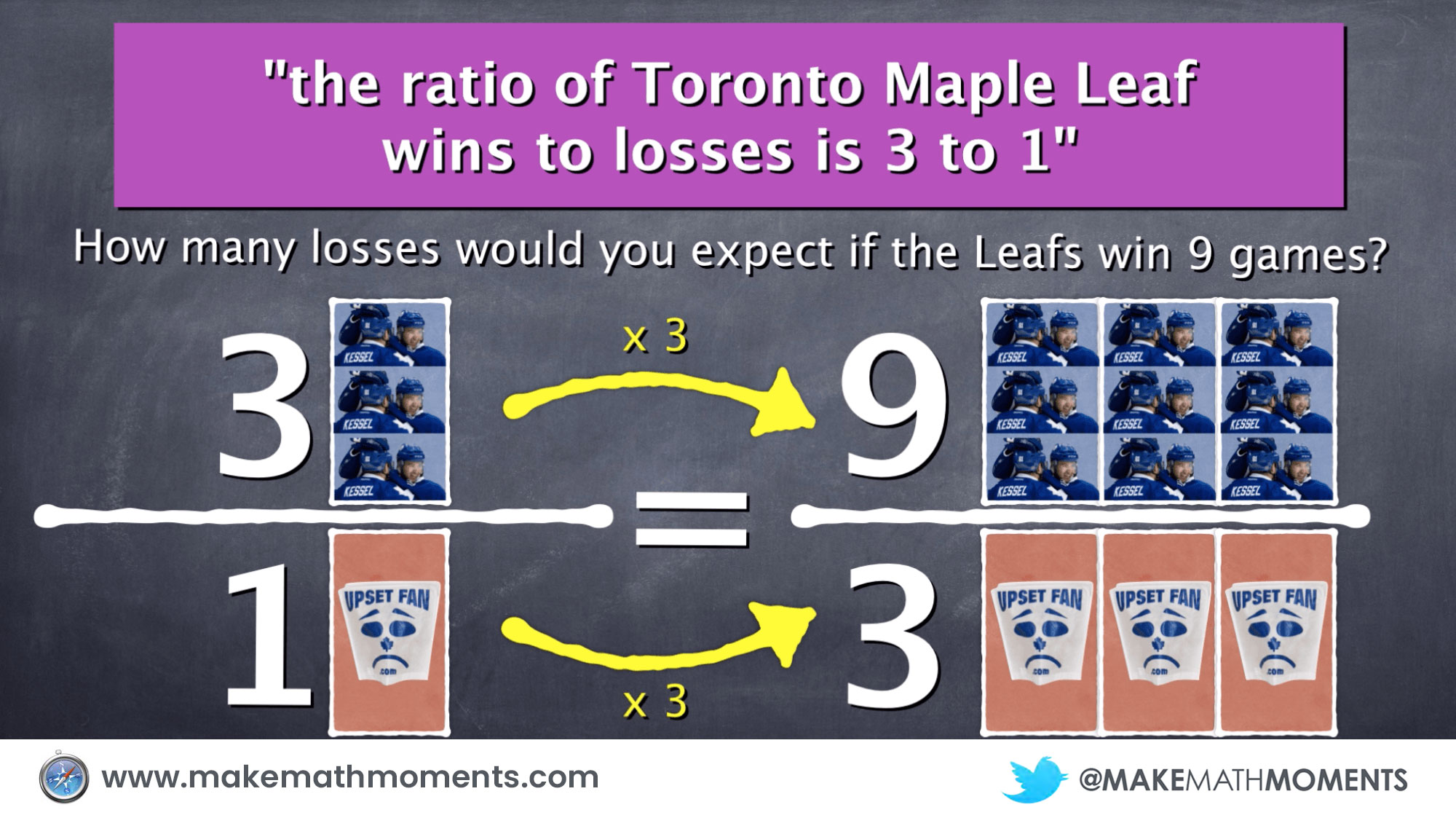In the same visual fashion, we solve a second problem and represent the 3:1 ratio in an obvious fashion.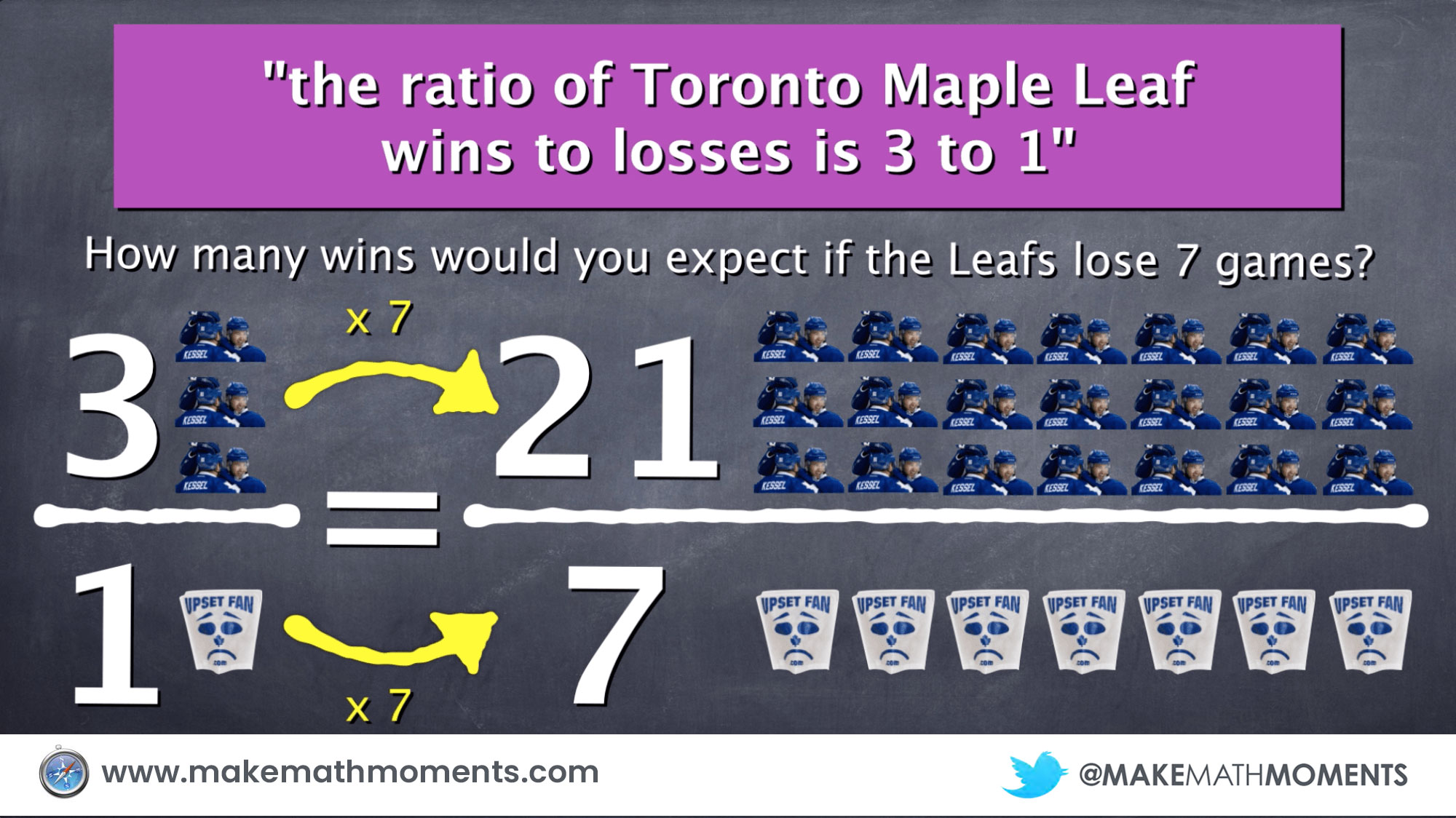We then move on to another example. This time, we change the ratio from 3:1 to 3:2 in order to scaffold students towards better understanding the factor the original ratio has been multiplied by.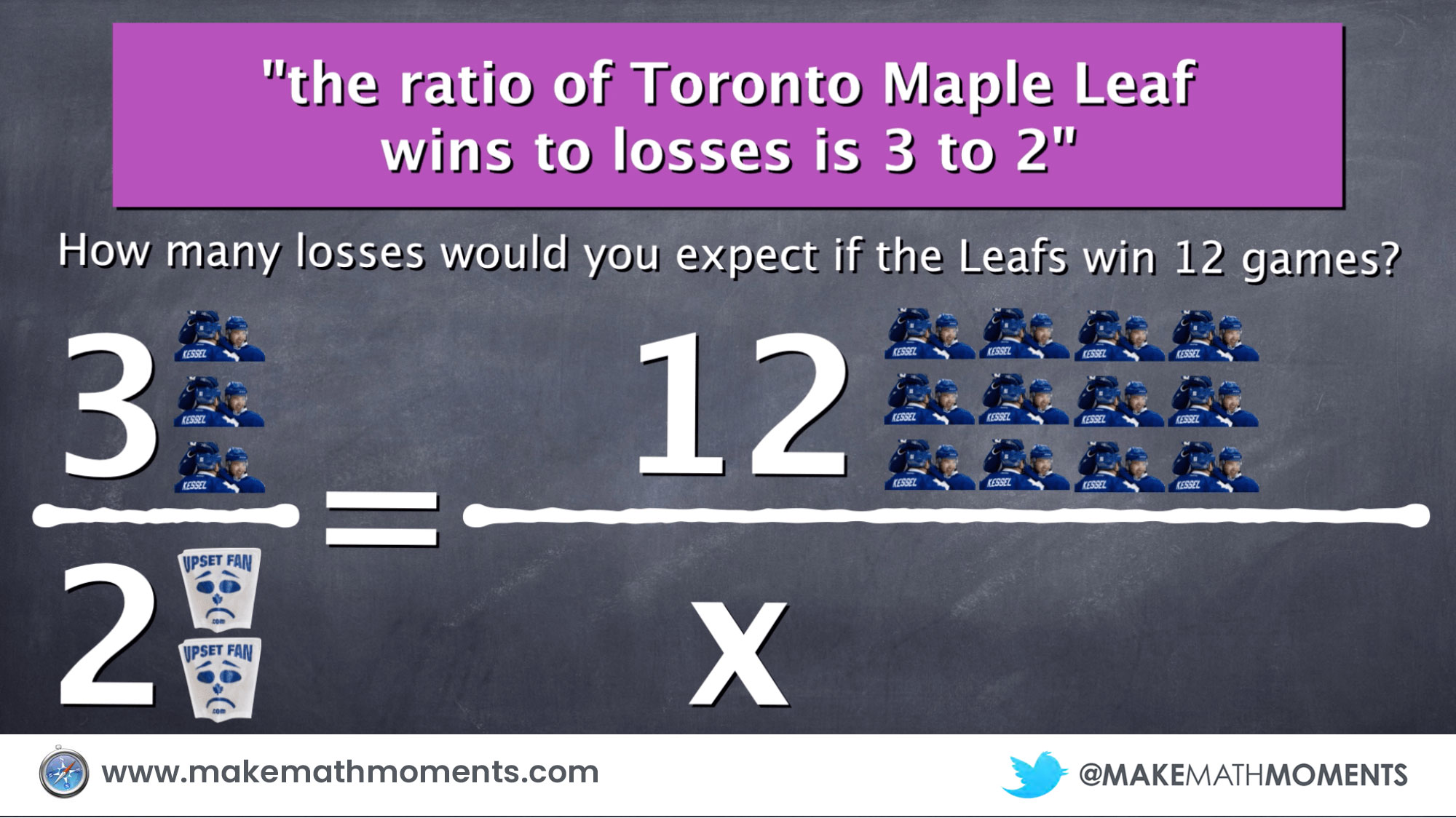We focus on the unknown factor or multiplier represented by the question mark (?) and work to find that unknown value. I use the concept of opposite operations (since we see 3 times a variable, we must divide by 3 to isolate the variable) and follow through by performing the same operation on the other side.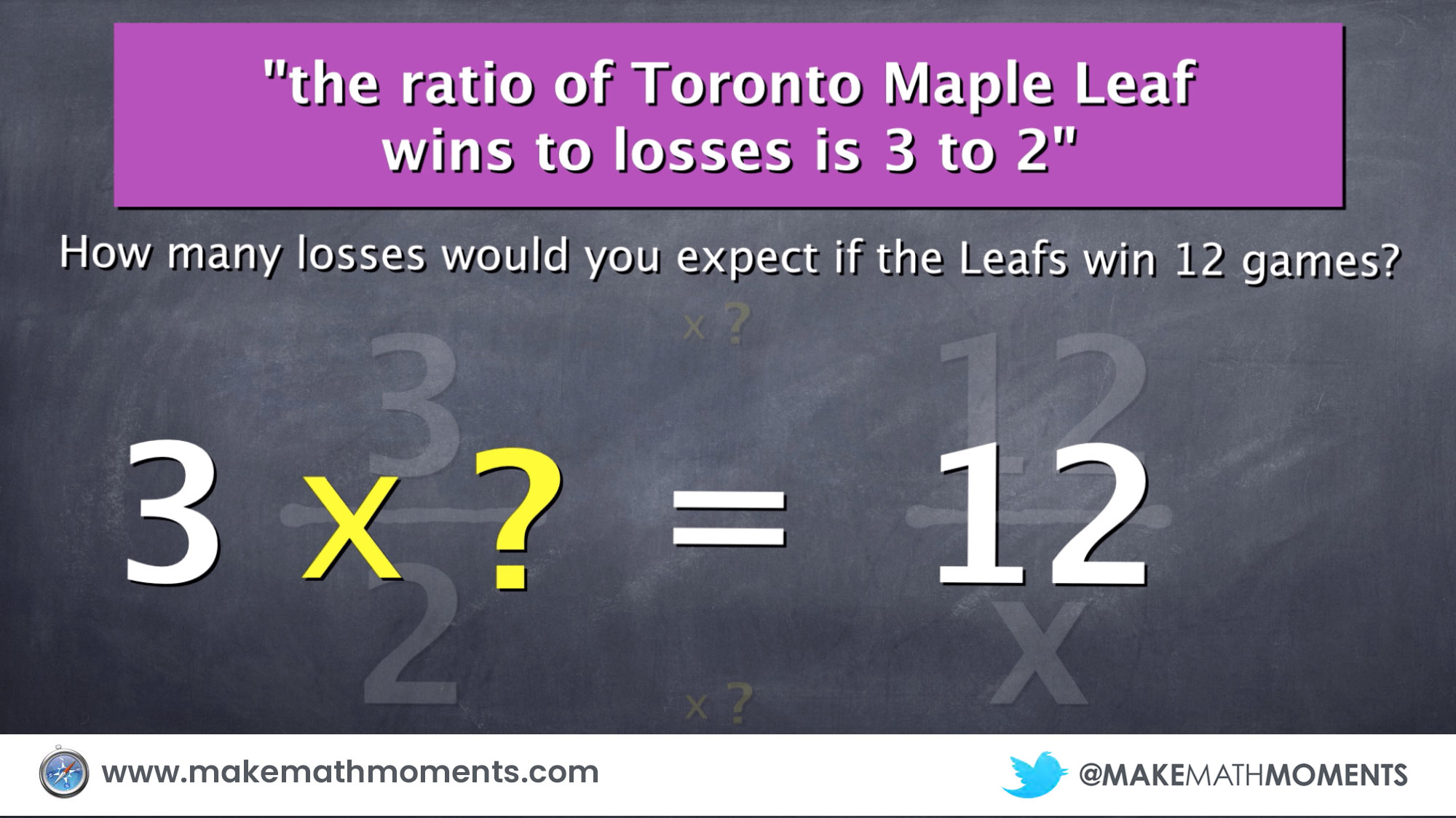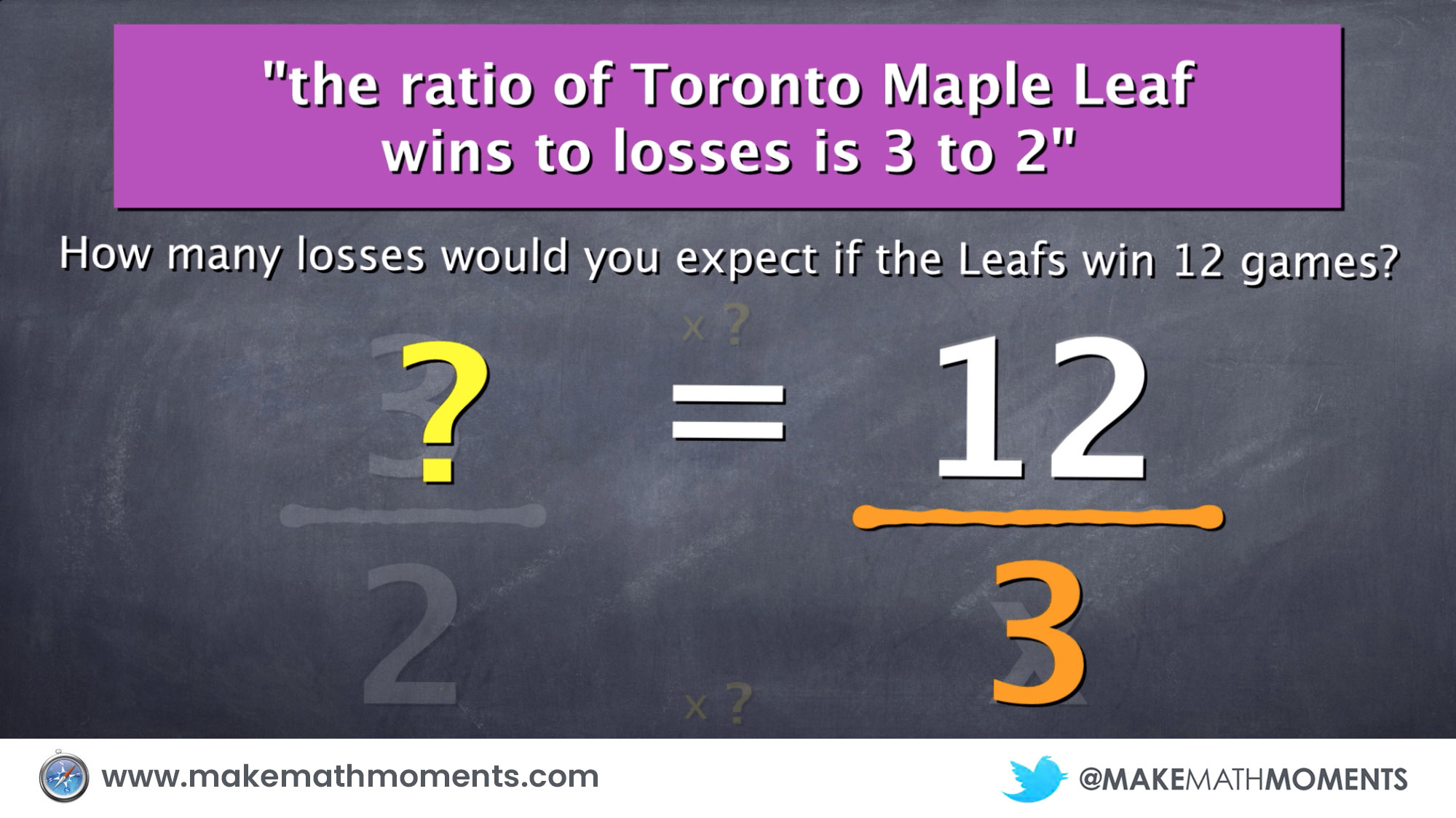We then use the factor/multiplier of 4 to determine the number of losses: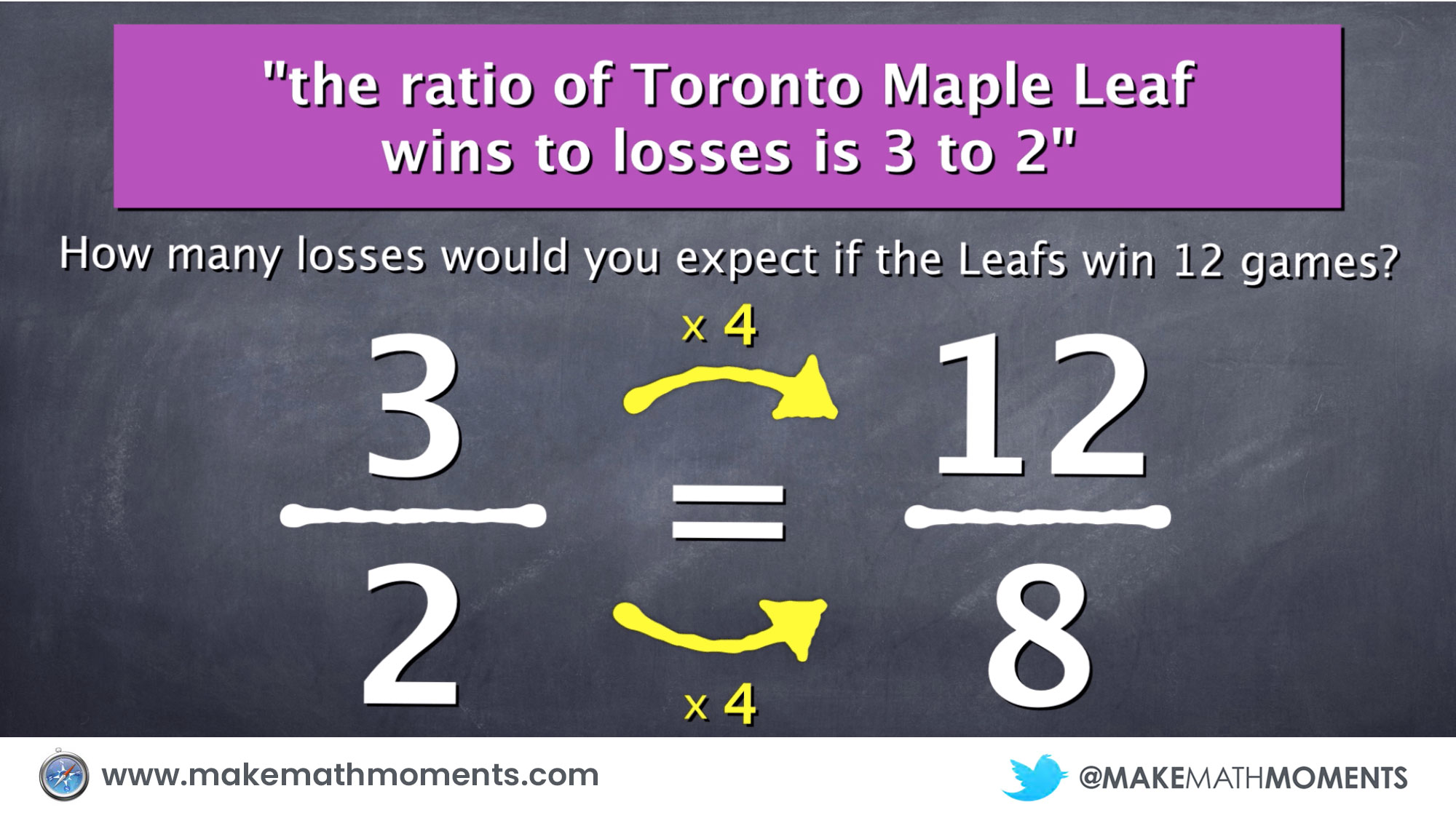To confirm our work, we bring the visuals back in.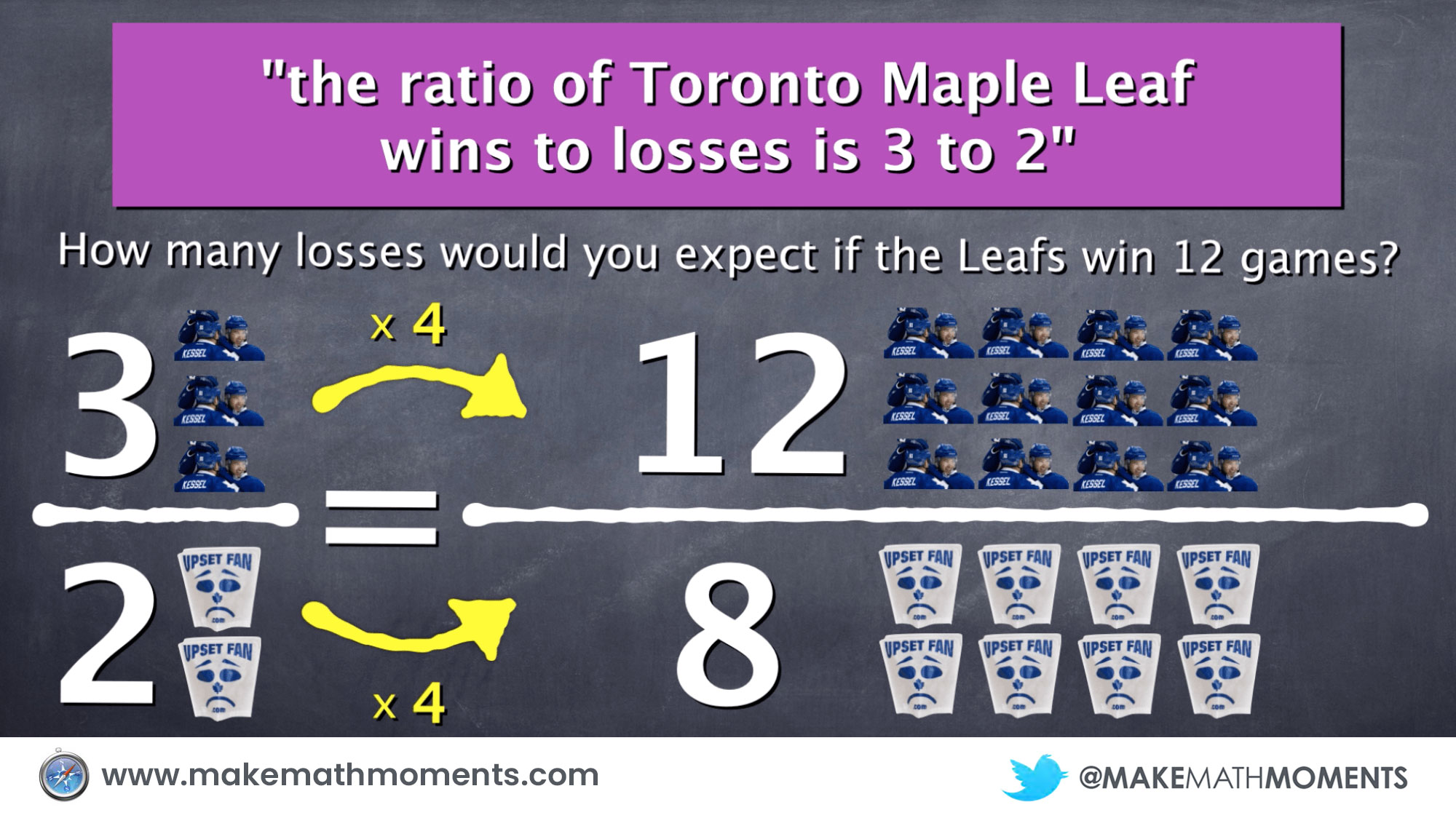Finally, we look at an example that makes using visuals very time consuming and inefficient. This drives home why using proportional reasoning to solve problems with ratios is important.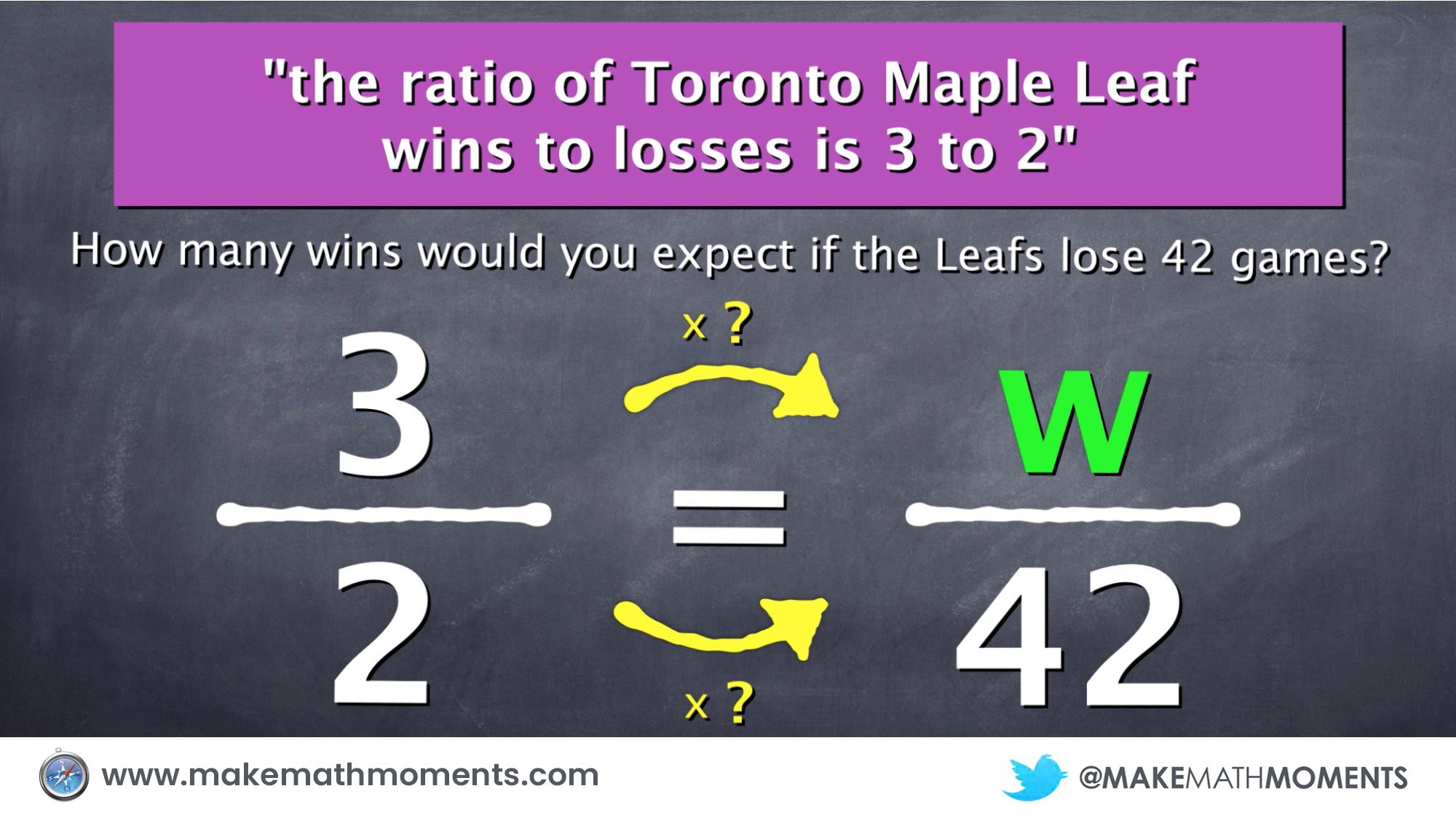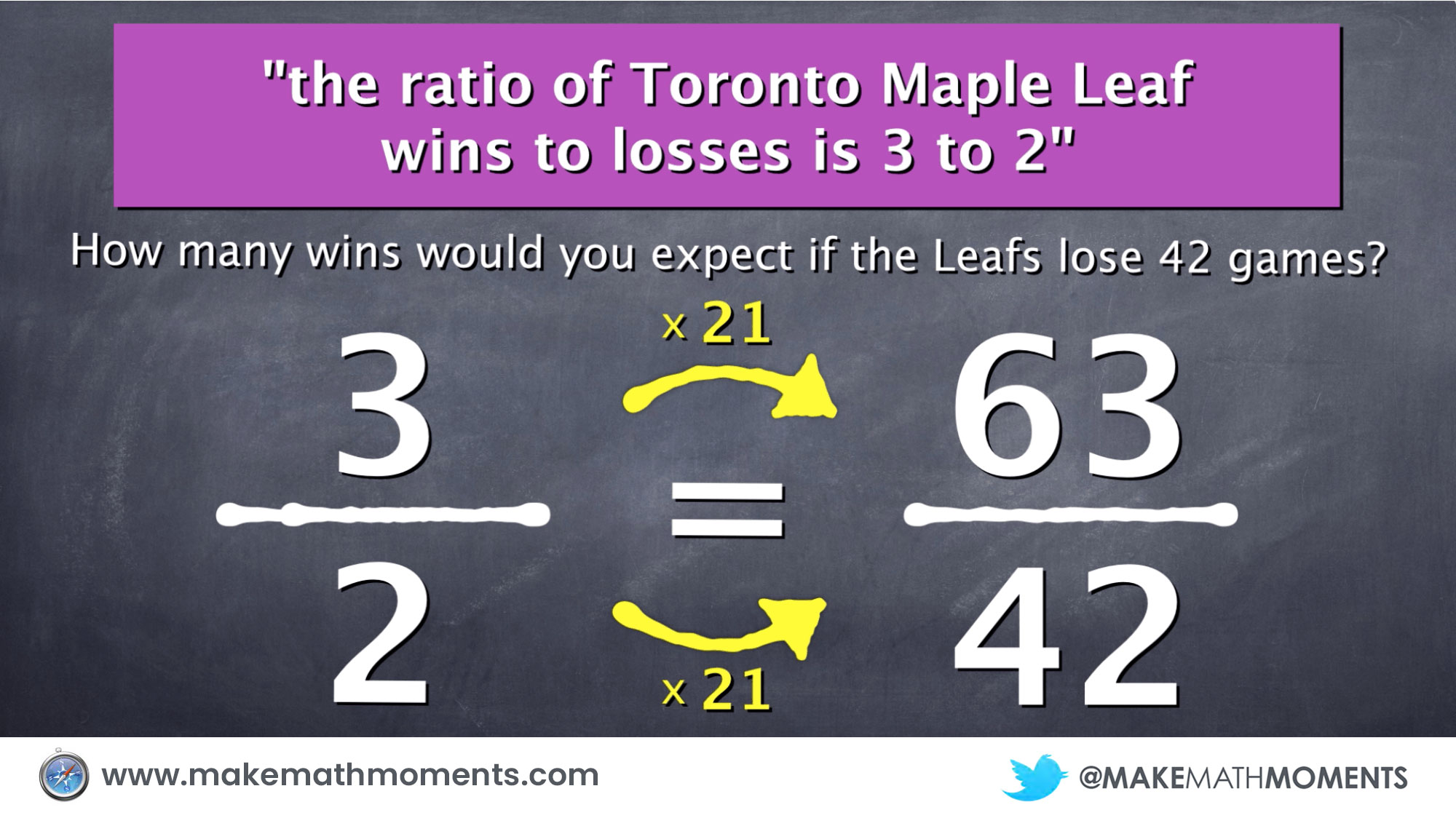As are all of my visual animations, they are my first attempt. Would appreciate any feedback (positive and negative) you can provide in the comments below.